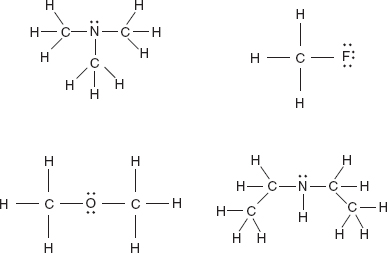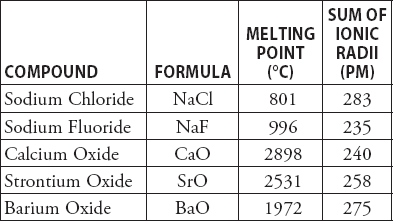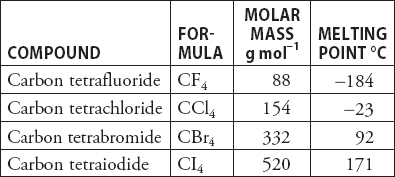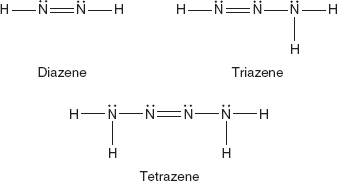# AP Chemistry Practice Test 45

### Test Information12 questions18 minutes

1. Oxidation of which of the following substances will yield a stronger acid?

2.Which of the compounds in the above diagram is capable of participating in hydrogen bonding?

3. Choose the reaction expected to have the greatest decrease in entropy.

4. A certain reaction is nonspontaneous under standard conditions, but becomes spontaneous at higher temperatures. What conclusions may be drawn under standard conditions?

5. Which of the following is the correct order of increasing acid strength?

`Questions 6-7 refer to the following information.Use the following information on the bases in the following diagram to answer questions.A large number of compounds adopt the sodium chloride structure. The following table contains some examples of the compounds in this group with their respective melting points and the sum of the cation-anion radii.`

6. Why are the melting points of the alkaline earth oxides (CaO, SrO, and BaO) so much higher than those of the other two compounds in the table?

7. Why do the melting points of the alkaline metal oxides decrease in the order CaO > SrO > BaO?

8. Joseph Priestly discovered oxygen gas by the decomposition of solid mercury(II) oxide, HgO, to oxygen gas, O2, and liquid mercury metal, Hg. How many moles of oxygen gas will form when 4.32 g of solid mercury(II) oxide decomposes? The formula mass of mercury(II) oxide is 216 g mol-1.

9.According to the data in the table above, which of the following best explains the trend in increasing melting points?

10. Determine the H+(aq) concentration in 1.0 M phenol, C6H5OH, solution. (The Ka for phenol is 1 × 10-10.)

11.Ammonia is the best-known nitrogen-hydrogen compound; however, there are a number of other nitrogen-hydrogen compounds, three of which are in the above diagram. Which of these has the longest average N-N bond length?

12. The above equilibrium was established in a 1.00 L container at a certain temperature. Once the system came to equilibrium, it was found that the following amounts of materials were present in the container:

(NH4)2CO3 = 8.00 moles, NH3 = 4.00 moles, CO2 = 2.00 moles, and H2O = 2.00 moles.

Determine the value of Kc at this temperature.